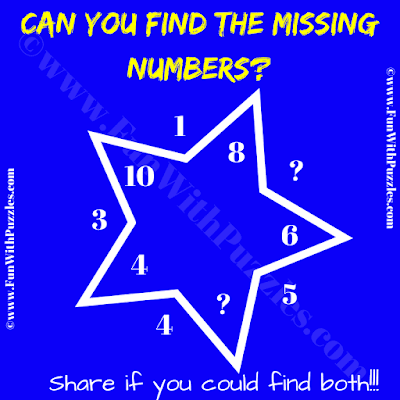It is a very interesting Math Picture Puzzle for school going students. In this Maths Picture Puzzle, you are given numbers inside and outside the star. Your challenge is to find the Maths relationship among these given numbers and then find the value of two missing numbers which will replace the given question marks. Can you solve this Math Picture Puzzle to find the values of both the missing numbers?Can you find the value of both the missing numbers?

The answer to this "Math Picture Puzzle for school students", can be viewed by clicking on the button. Please do give your best try before looking at the answer.